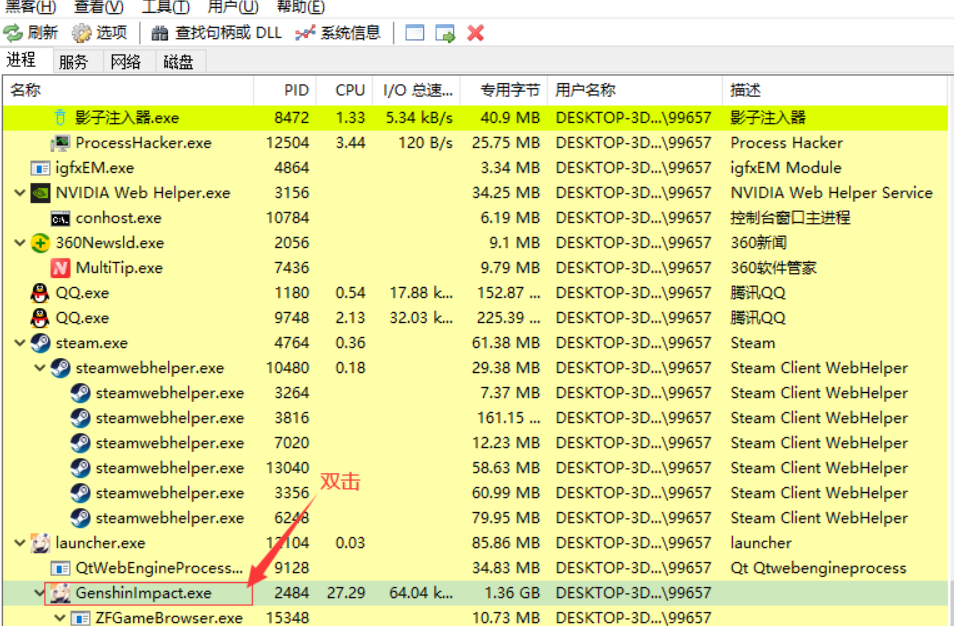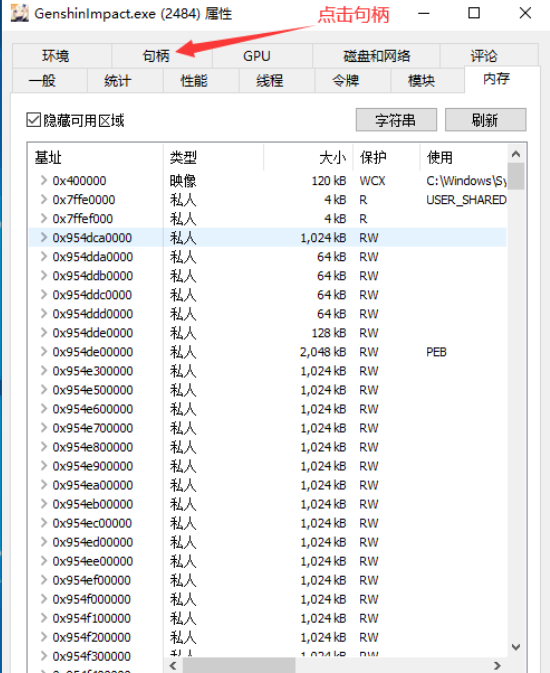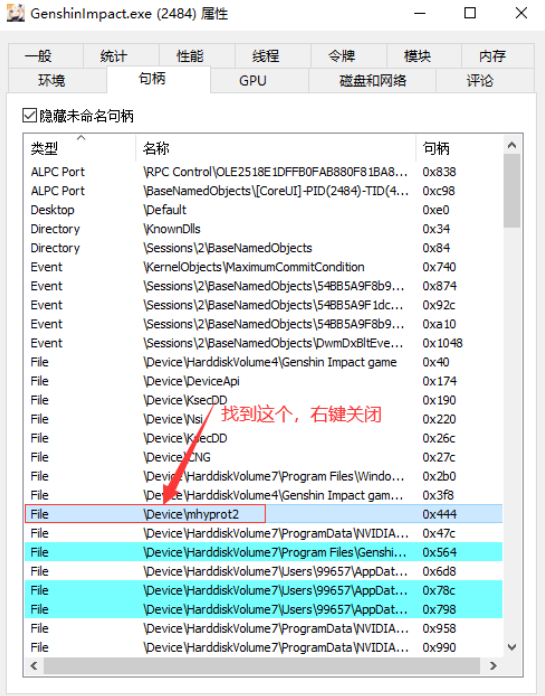﻿ 原神·老外五项多功能修改器国服版v2.2 请看完全版教程 - 科技岛## 程序功能

F1=无视敌人攻击
F2=无限[E/Q]
F3=无限耐力
F5=敌人无视玩家
F6=解锁FPS
F7=移动加速
F8=总是看见

~=自动掠夺 [F]
1+2=杀死附近的敌人
Q+1=生成敌人
Q+2=删除敌人
1+3=选择激活到1
1+4=选择激活到0
2+3=破坏附近的物品
2+4=选择激活到2
2+5=秒蓄力
3+4=开启修改伤害
3+5=设置伤害成1500
3+6=设置伤害成2000
3+7=设置伤害成3000
4+5=世界扭曲设置成2
4+6=世界扭曲设置成1
W+1=无敌模式【内置无限技能，无限耐力，总是看见，伤害修改】
3+5=设置无敌模式内置伤害成100
3+6=设置无敌模式内置伤害成500
3+7=设置无敌模式内置伤害成1000

## 使用介绍

1.启动游戏加入游戏，管理员权限运行进程黑客工具

2.找到游戏进程，并双击

## 教程截图## 游戏截图## 文章评论2021-12-05 01:57 3楼2021-11-27 20:09 2楼2021-11-27 21:08
@c：等国外网站更新了就给你搞过来！2021-11-25 08:57 1楼